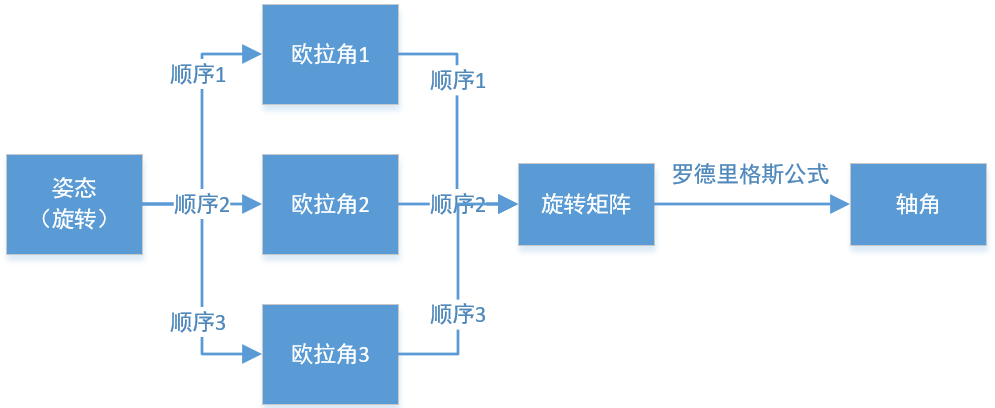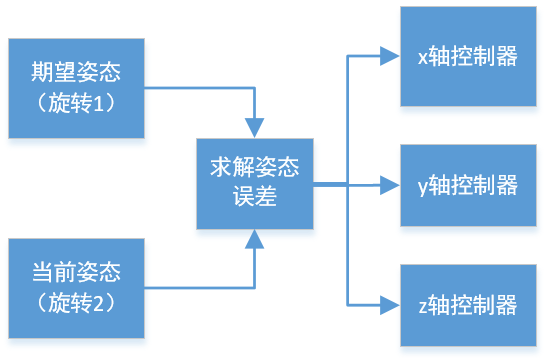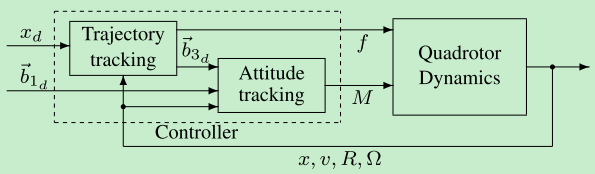# 【飞控】姿态误差 (二)-旋转矩阵做差zyx顺序的欧拉角
[-1.04537914205813,-0.0795839823878026,0.909278065160895]
xyz顺序的欧拉角
[0.523636366815730,-0.785388573397448,-0.785398163397448]

[0.5000 -0.8624 0.0795;
0.5000 0.3624 0.7866;
-0.7071 -0.3536 0.6124]

(旋转矩阵描述的是个状态，它无法描述运动过程。)

$$C_a^b$$

$$A_b=C_a^bA_a$$

$$A_2=C_1^2A_1$$

$$A_3=C_2^3A_2$$

$$A_3=C_2^3C_1^2A_1$$

$$C_1^3=C_{\bcancel{2}}^3C_1^{\bcancel{2}}$$

$${C_2^3}^{-1}C_1^3=C_1^2$$

$${C_2^3}^{T}C_1^3=C_1^2$$

\begin{aligned} {C_2^3}^{T}C_1^3&=C_1^2 \\ {C_3^2}C_1^3&=C_1^2 \\ C_3^2&={C_2^3}^{T} \end{aligned}

1.描述与顺序无关的一次旋转。
2.可以描述空间中所有的姿态。
2.做差之后依然是个旋转.
3.做差的运算非常简单，只涉及矩阵乘法和矩阵转置。

PS: *不是所有的33矩阵都是旋转矩阵，旋转矩阵有两个约束：正交且行列式=1**，即：

$$CC^T=1, \det C=1$$1: 是将旋转矩阵转换成一个三个参数的描述方法(当然不是欧拉角)。
2: 设计一个基于旋转矩阵的控制器。尤其是位置外环直接得到期望旋转矩阵，相当于由里到外重新写一套代码了！